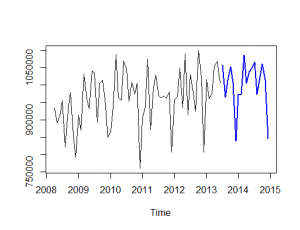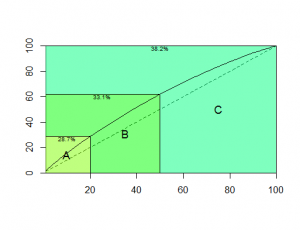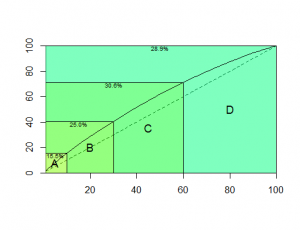# Update for TStools

By | May 4, 2014

Amongst various minor improvements a few interesting new functions have been added to TStools package for R:

• Theta method
• ABC-XYZ analysis

Theta method was found to be the most accurate in the M3 forecasting competition, but since then there has been limited use of the method. As it was later shown, the M3 competition Theta method setup is equivalent to using a single exponential smoothing with drift and an additive constant, with classical decomposition.

This is a beta function incorporating a few tweaks over the original method:

• Decomposition is performed only if seasonality is detected. The original method tested this using an ACF based test. This implementation uses the approach used in the seasplot function;
• Seasonality can be additive or multiplicative, estimated using classical decomposition or a pure seasonal exponential smoothing model;
• If decomposition is performed then the Theta0 line is based on the trend component, rather than the deseasonalised time series;
• Theta0 is a linear trend only if changes in the level are detected, otherwise it is just a mean level estimation;
• Theta0 and Theta2 lines, as well as the pure seasonal model can be optimised with various cost functions. The idea is that it may be beneficial to have robust Theta0 line (optimised on MAE) and more reactive Theta2 line and so on.

Limited benchmarking has shown that this implementation is marginally more accurate than the original Theta method, or the reformulated one. The best setup and options are still under investigation!

To use the method simply run:

`> theta(referrals,h=18)`

The output includes the forecasted values, the result of trend and seasonality identification, the predicted values of the various Theta lines and the seasonal component, model parameters and cost functions used. There are two different visualisations available, just the forecast:or the forecast with the Theta lines. I quite prefer the second one, as it explains how the forecast is calculated:There are three functions to support the ABC-XYZ analysis. Let us first get some quarterly time series to analyse:

```> library(Mcomp)
> M3.subset <- subset(M3, "QUARTERLY")
> x <- matrix(NA,46,100)
> for (i in 1:100){
x.temp <- c(M3.subset[[i]]\$x,M3.subset[[i]]\$xx)
x[1:length(x.temp),i] <-x.temp
}```

To perform ABC analysis use the abc function. Unless the importance of a series is calculated externally, then it is estimated as the mean volume of sales of a product.

`> imp <- abc(x,outplot=TRUE)`The plot shows how concentrated is the value in the A, B or C classes. The bigger the difference between the straight diagonal line and the concentration curve, the more value is included in the A and B classes. For example, here we have set class A to contain 20% of the products, but it accounts for 28.7% of the value. Another example with very high concentration is the following:Here A products account for 47.9% of the total value. On the other hand, the C products account for only 11.8% of the total value, even though this class contains 50% of the items.

Although ABC analysis usually classifies items in three groups, it is fairly easy to introduce more groups, depending on the percentages used:

`> abc(x,c(0.1,0.2,0.3,0.4))`Similarly XYZ analysis can be done using the xyz function. Forecastability can be estimated in three different ways. The default is to calculate a scaled in-sample RMSE of the best of two methods: Naive and Seasonal Naive. The scaling is done by dividing the RMSE with mean of each time series. The second approach is to calculate the coefficient of variation for each time series. The last approach is to fit an ETS model (using the forecast package) and calculate again the scaled in-sample RMSE. The advantage of the first approach is that it can tackle with seasonal time series and it is relatively fast. The second approach is very simple, but it does not handle well trended or seasonal time series. The last approach selects that appropriate ETS model for each time series, thus being able to handle most types of time series. On the downside, it requires substantially more time.

`> frc <- xyz(x,m=4,outplot=TRUE)`As with the abc function, external measures of forecastability can be used and any number of classes.

Finally, the function the abcxyz visualises the combined classification:

```> abcxyz(imp,frc)
Z  Y  X
A  4  7  9
B  6  7 17
C 10 16 24
```

## 2 thoughts on “Update for TStools”

1.Manuel

Dear Nikolaos,

I was experimenting with your Theta model implementation, but I obtain a “extrange” values for h=1 with next simulated time series:

trend <- ts(c(1:72), freq=12)
seasonal <- ts(rep(c(1:12), 6), freq=12)
S <- trend + seasonal

theta(S)\$frc

However,

theta(seasonal)\$frc fit a perfect forecast and theta(trend)\$frc fit a cuasi-perfect forecast

Is this a limitation of Theta model or a "bug" in the implementation.?

Many thanks,

1.Nikos Post author

Hi Manuel,

Interesting simulation. The problem is in the time series decomposition. The original Theta was proposed assuming a multiplicative decomposition and is implemented here as the default option.